Create a new printableMath Worksheets

Sample - Click above to make a new math worksheet (PDF).
 Name _____________________________Date ___________________
Subtraction
Write the difference.

1.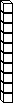-4 tens  -  1 ten

=  ___ tens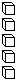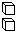-7 ones  -  5 ones

=  ___ ones

47  -  15  =  ______
2.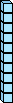-7 tens  -  1 ten

=  ___ tens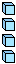-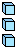4 ones  -  3 ones

=  ___ ones

74  -  13  =  ______
 3 * This is a pre-made sheet.Use the link at the top of the page for a printable page.
4.-4 tens  -  4 tens

=  ___ tens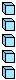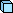-6 ones  -  1 one

=  ___ ones

46  -  41  =  ______
5.-8 tens  -  2 tens

=  ___ tens-2 ones  -  2 ones

=  ___ ones

82  -  22  =  ______
6.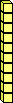-7 tens  -  2 tens

=  ___ tens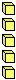-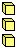5 ones  -  3 ones

=  ___ ones

75  -  23  =  ______